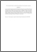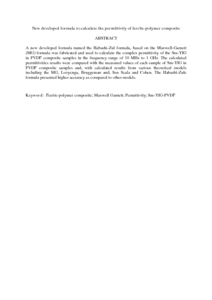# New developed formula to calculate the permittivity of ferrite-polymer composite

## Citation

Al-Habashi, Ramadan and Abbas, Zulkifly (2013) New developed formula to calculate the permittivity of ferrite-polymer composite. Key Engineering Materials, 553. pp. 53-58. ISSN 1013-9826

## Abstract

A new developed formula named the Habashi-Zul formula, based on the Maxwell-Garnett (MG) formula was fabricated and used to calculate the complex permittivity of the Sm-YIG in PVDF composite samples in the frequency range of 10 MHz to 1 GHz. The calculated permittivities results were compared with the measured values of each sample of Sm-YIG in PVDF composite samples and, with calculated results from various theoretical models including the MG, Looyenga, Bruggeman and, Sen Scala and Cohen. The Habashi-Zule formula presented higher accuracy as compared to other models.Preview
PDF (Abstract)
New developed formula to calculate the permittivity of ferrite.pdfView Item